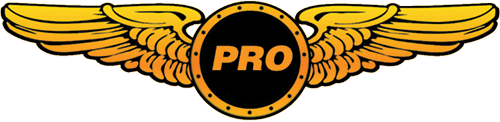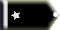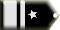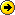# Vapour trails messed up?!deanos63 Trainee

I have white lines falling from behind planes to the ground.

let me explain,

when a ai plane or my own plane get to a certain altitude the vapour trails appear, but also with the vapour trails there is a near vertical set of vapour trails that fall all the way to the ground which then move with the aircraft. I will be sitting waiting to taxi at a gate and i will see these lines appear, that when i know that a plane is passing over head.

anyone else experience this before?deanos63 Trainee

i'll try and get a screen capture of it when it happens next.

its a bit weird i don;t know if it has somethig to to do with the files in the effects folder.deanos63 Trainee

i have a great pic of what i mean how can i show it?Greekman72 Chief Captain

Read thisdeanos63 Trainee

ah coolio!

heres what i mean

look at the drop away of the trail

differnet angle?

what would the files be to delet the vapour trails? if i remove them one by one maybe i can find out the cause?

oh by the way thanks for showing me how to upload pics!deanos63 Trainee

when i removed the file, fx_contrail_l, when i remived it everything dissappeared, so i don't know if it is a problem with this file or not?

heres what it says when i open it in notepad

[Library Effect]
Version=1.00

[Emitter.0]
Delay=0.30, 0.32
Bounce=0.00
Rate=1.00, 1.01
X Emitter Velocity=0.00, 0.00
Y Emitter Velocity=0.00, 0.00
Z Emitter Velocity=0.00, 0.00
Drag=0.00, 0.01
X Particle Velocity=0.00, 0.00
Y Particle Velocity=0.00, 0.00
Z Particle Velocity=0.00, 0.00
X Rotation=0.00, 0.00
Y Rotation=0.00, 0.00
Z Rotation=0.00, 0.00
X Offset=0.00, 0.00
Y Offset=0.00, 0.00
Z Offset=0.00, 0.00

[Particle.0]
Type=21
X Scale=10.00, 10.00
Y Scale=10.00, 10.00
Z Scale=0.00, 0.00
X Scale Rate=0.25, 0.30
Y Scale Rate=0.25, 0.30
Z Scale Rate=0.00, 0.00
Drag=-2.60, -1.79
Color Rate=0.00, 0.00
X Offset=0.00, 0.00
Y Offset=0.00, 0.00
Z Offset=0.00, 0.00
Face=0, 0, 0

[ParticleAttributes.0]
Blend Mode=1
Texture=fx_smoke.bmp
Bounce=0.00
Color Start=255, 255, 255, 200
Color End=249, 249, 249, 255
Jitter Distance=1.00
Jitter Time=5.00
TempK=109.00
TempRate=0.01
uv1=0.00, 0.00
uv2=1.00, 1.00
X Scale Goal=10.00
Y Scale Goal=10.00
Z Scale Goal=0.00
Extrude Length=200.00
Extrude Pitch Max=2.00

[Emitter.1]
Delay=0.00, 0.00
Bounce=0.00
Rate=20.00, 30.00
X Emitter Velocity=0.00, 0.00
Y Emitter Velocity=0.00, 0.00
Z Emitter Velocity=0.00, 0.00
Drag=0.00, 0.00
X Particle Velocity=0.00, 0.00
Y Particle Velocity=0.00, 0.00
Z Particle Velocity=0.00, 0.00
X Rotation=0.00, 0.00
Y Rotation=0.00, 0.00
Z Rotation=0.00, 0.00
X Offset=0.00, 0.00
Y Offset=0.00, 0.00
Z Offset=0.00, 0.00

[Particle.1]
Type=19
X Scale=3.00, 6.00
Y Scale=3.00, 6.00
Z Scale=0.00, 0.00
X Scale Rate=0.50, 1.00
Y Scale Rate=0.50, 1.00
Z Scale Rate=0.00, 0.00
Drag=-4.00, -3.98
Color Rate=0.33, 0.45
X Offset=0.00, 0.00
Y Offset=0.00, 0.00
Z Offset=0.00, 0.00
Rotation=-60.00, 60.00
Face=1, 1, 1

[ParticleAttributes.1]
Blend Mode=1
Texture=fx_1.bmp
Bounce=0.00
Color Start=255, 255, 255, 45
Color End=228, 231, 233, 0
Jitter Distance=0.20
Jitter Time=1.00
TempK=107.00
TempRate=0.01
uv1=0.50, 0.75
uv2=0.75, 1.00
X Scale Goal=1.00
Y Scale Goal=1.00
Z Scale Goal=0.00
Extrude Length=0.00
Extrude Pitch Max=0.00

[Emitter.2]
Delay=0.00, 0.00
Bounce=0.00
Rate=1.00, 1.01
X Emitter Velocity=0.00, 0.00
Y Emitter Velocity=0.00, 0.00
Z Emitter Velocity=0.00, 0.00
Drag=0.00, 0.00
X Particle Velocity=0.00, 0.00
Y Particle Velocity=0.00, 0.00
Z Particle Velocity=0.00, 0.00
X Rotation=0.00, 0.00
Y Rotation=0.00, 0.00
Z Rotation=0.00, 0.00
X Offset=0.00, 0.00
Y Offset=0.00, 0.00
Z Offset=0.00, 0.00

[Particle.2]
Type=21
X Scale=15.00, 15.00
Y Scale=15.00, 15.00
Z Scale=0.00, 0.00
X Scale Rate=0.15, 0.15
Y Scale Rate=0.15, 0.15
Z Scale Rate=0.00, 0.00
Drag=0.00, 0.00
Color Rate=0.00, 0.00
X Offset=0.00, 0.00
Y Offset=0.00, 0.00
Z Offset=0.00, 0.00
Face=0, 0, 0

[ParticleAttributes.2]
Blend Mode=1
Texture=fx_smoke.bmp
Bounce=0.00
Color Start=250, 250, 253, 200
Color End=252, 252, 252, 0
Jitter Distance=0.00
Jitter Time=0.00
uv1=0.00, 0.00
uv2=1.00, 1.00
X Scale Goal=20.00
Y Scale Goal=20.00
Z Scale Goal=0.00
Extrude Length=500.00
Extrude Pitch Max=5.00